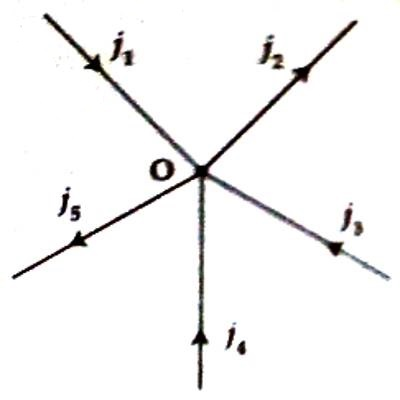# Kirchoff’s First Law: The Current Law

Kirchoff’s First Law: The Current Law

First law: In any electrical network, the algebraic sum of currents meeting at a junction is always zero. This law is known as the current law.

Kirchhoff’s Current Law or KCL, states that the “total current or charge entering a junction or node is exactly equal to the charge leaving the node as it has no other place to go except to leave, as no charge is lost within the node“. In other words, the algebraic sum of ALL the currents entering and leaving a node must be equal to zero, I(exiting) + I(entering) = 0. This idea by Kirchhoff is commonly known as the Conservation of Charge.

Explanation: Suppose at point 0 in a circuit five currents i1, i2, i3, i4 and i5 of 1 different direction have met [Figure].Normally, the current flowing towards the junction is taken as positive, while current flowing out from the junction is taken as negative. So, applying Kirchhoff’s law on the currents meeting at junction O, we get,

i1, i3, i4 – i2 – i5 = 0

or, i1, i3, i4 + (– i2) + (– i5) = 0

We know, current is nothing but the flow of charges. Now, if the sum of currents at the junction is not zero means the creation or destruction of charges at the junction – which is totally against the conservation principle of charges. So, nowhere in the circuit charges can be stored. Symbolically or mathematically the law can be expressed as, Σi = 0.